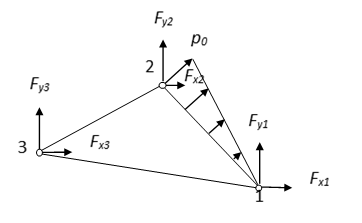# 第八章第四节测试题【含答案】 有限元分析及应用

（1）单选题

A  是

B  否

（2）单选题A  $0$

B  $\frac{1}{6}{p_0}t({y_2} - {y_1})$

C  $\frac{1}{3}{p_0}t({y_2} - {y_1})$

D  $\frac{2}{3}{p_0}t({y_2} - {y_1})$

（3）单选题

A  是

B  否